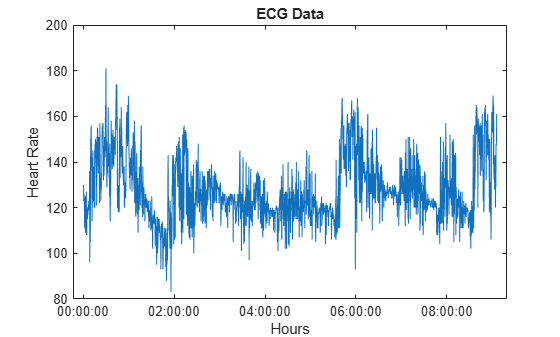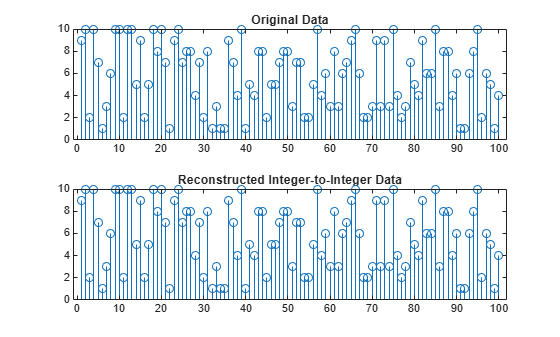# ihaart

Inverse 1-D Haar wavelet transform

## Syntax

``xrec = ihaart(a,d)``
``xrec = ihaart(a,d,level)``
``xrec = ihaart(___,integerflag)``

## Description

example

````xrec = ihaart(a,d)` returns the inverse 1-D Haar transform, `xrec`, for the approximation coefficients, `a`, and the wavelet coefficients, `d`. Both `a` and `d` are obtained from `haart`. ```

example

````xrec = ihaart(a,d,level)` returns the inverse 1-D Haar transform at the specified level. ```

example

````xrec = ihaart(___,integerflag)` specifies how the inverse 1-D Haar transform handles integer-valued data, using any of the previous syntaxes.```

## Examples

collapse all

Obtain the Haar and inverse Haar transforms of noisy data.

` load noisdopp;`

Obtain the Haar transform of the noisy signal.

` [a,d] = haart(noisdopp);`

Reconstruct the data by inverting the Haar transform.

` xrec = ihaart(a,d);`

Compare the original and reconstructed data by determining the maximum difference between them. The difference is essentially zero, which indicates a near-perfect reconstruction.

` max(abs(xrec-noisdopp'))`
```ans = 4.4409e-15 ```

Obtain the Haar transform and inverse Haar transform of ECG heart rate data.

Load and plot the ECG data.

```load BabyECGData; plot(times,HR) xlabel('Hours') ylabel('Heart Rate') title('ECG Data')```Obtain the Haar transform and inverse Haar transform. Compare the reconstructed data at level 4 to the original data.

```[a,d] = haart(HR); HaarHR = ihaart(a,d,4); figure plot(times,HaarHR) xlabel('Hours') ylabel('Heart Rate') title('Haar Approximation of Heart Rate')```Obtain the Haar and inverse Haar transforms for a series of random integers.

Create the series.

`x = randi(10,100,1);`

Obtain the Haar and inverse Haar transforms.

```[a,d] = haart(x,'integer'); xrec = ihaart(a,d,'integer');```

Plot and compare the original and reconstructed data.

```subplot(2,1,1) stem(x); title('Original Data') subplot(2,1,2) stem(xrec) title('Reconstructed Integer-to-Integer Data')```Determine the maximum difference between the original data values and the reconstructed values. The difference is zero, which indicates perfect reconstruction.

`max(abs(x(:)-xrec(:)))`
```ans = 0 ```

## Input Arguments

collapse all

Approximation coefficients, specified as a scalar, vector, or matrix of coefficients, depending on the level to which the Haar transform was calculated. `a` is an output from the `haart` function.

Approximation, or scaling, coefficients are a lowpass representation of the input. At each level the approximation coefficients are divided into coarser approximation and detail coefficients.

Data Types: `single` | `double`
Complex Number Support: Yes

Detail coefficients, specified as a scalar, vector, matrix, or cell array of wavelet coefficients. `d` is an output from the `haart` function. The number of detail coefficients depends on the selected level and the length of the input. If `d` is a cell array, the elements of `d` are ordered from finest to coarsest resolution.

If `d` is a cell array, it can contain scalars, vectors, or matrices. The level of the Haar transform equals the number of elements in `d`.

If `d` is a vector or matrix, the Haar transform was computed only down to one level coarser in resolution.

If `a` and the elements of `d` are vectors, `xrec` is a vector. If `a` and the elements of `d` are matrices, `xrec` is a matrix, where each column is the inverse Haar transform of the corresponding columns in `a` and `d`.

Data Types: `single` | `double`
Complex Number Support: Yes

Maximum level to which to invert the Haar transform, specified as a nonnegative integer. If `d` is a cell array, `level` is less than or equal to `length(d)-1`. If `d` is a vector or matrix, `level` must equal `0` or be unspecified. The level must be less than the level used to obtain `a` and `d` from `haart`.

Integer-valued data handling, specified as either `'noninteger'` or `'integer'`. `'noninteger'` does not preserve integer-valued data, and `'integer'` preserves it. The `'integer'` option applies only if all elements of `a` and `d` are integer-valued. You must have used `'integer'` with `haart` to obtain integer-valued `a` and `d` inputs. The inverse 1-D Haar transform algorithm, however, uses floating-point arithmetic.

## Output Arguments

collapse all

Inverse 1-D Haar wavelet transform, returned as a vector or matrix. If `a` and the elements of `d` are vectors, `xrec` is a vector. If `a` and the elements of `d` are matrices, `xrec` is a matrix, where each column is the inverse 1-D Haar transform of the corresponding columns in `a` and `d`.

Data Types: `single` | `double`

## Extended Capabilities

### GPU ArraysAccelerate code by running on a graphics processing unit (GPU) using Parallel Computing Toolbox™.